Скачать презентацию CS 290 N 219 Sparse Matrix Algorithms

1c7e51204b883138700c325758022283.ppt

• Количество слайдов: 13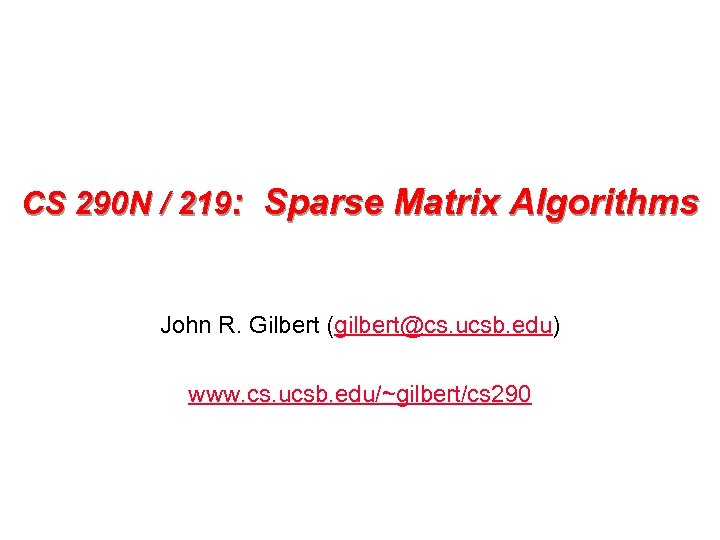CS 290 N / 219: Sparse Matrix Algorithms John R. Gilbert ([email protected] ucsb. edu) www. cs. ucsb. edu/~gilbert/cs 290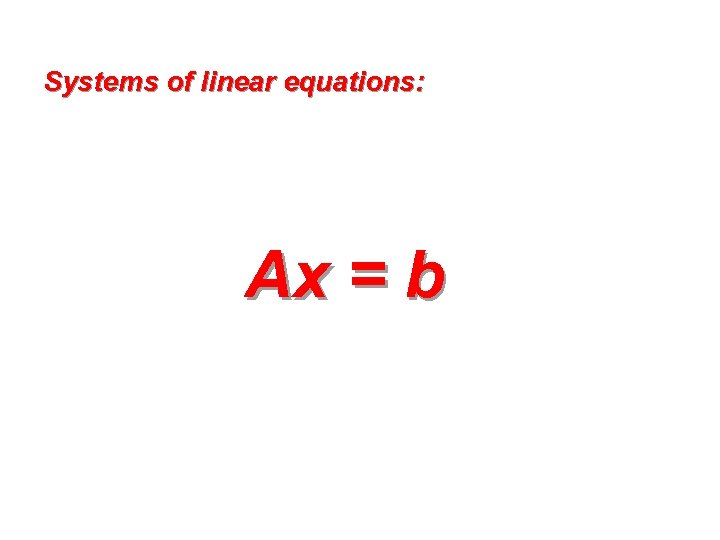Systems of linear equations: Ax = b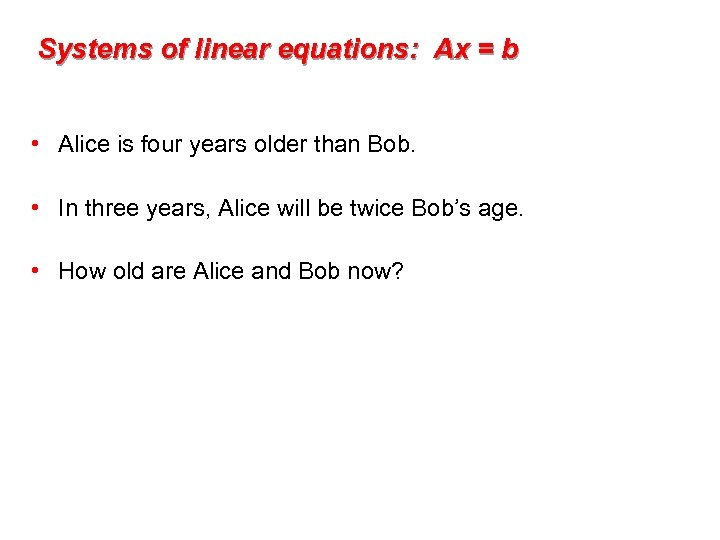Systems of linear equations: Ax = b • Alice is four years older than Bob. • In three years, Alice will be twice Bob’s age. • How old are Alice and Bob now?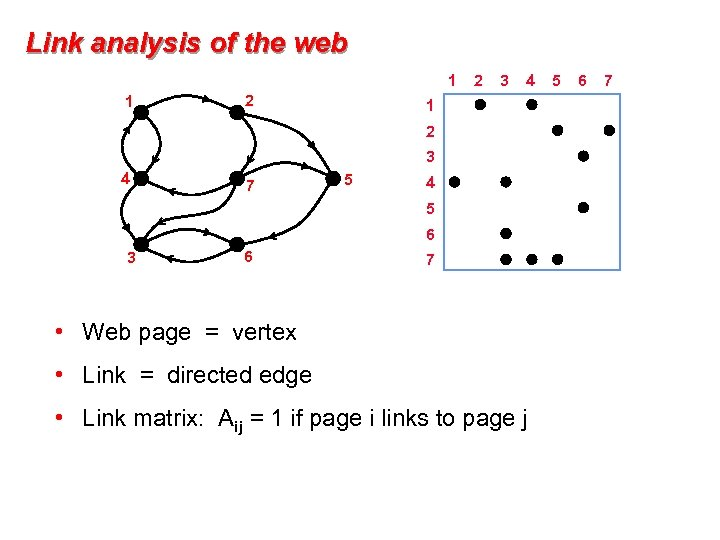Link analysis of the web 1 1 2 2 3 4 1 2 3 4 7 5 4 5 6 3 6 7 • Web page = vertex • Link = directed edge • Link matrix: Aij = 1 if page i links to page j 5 6 7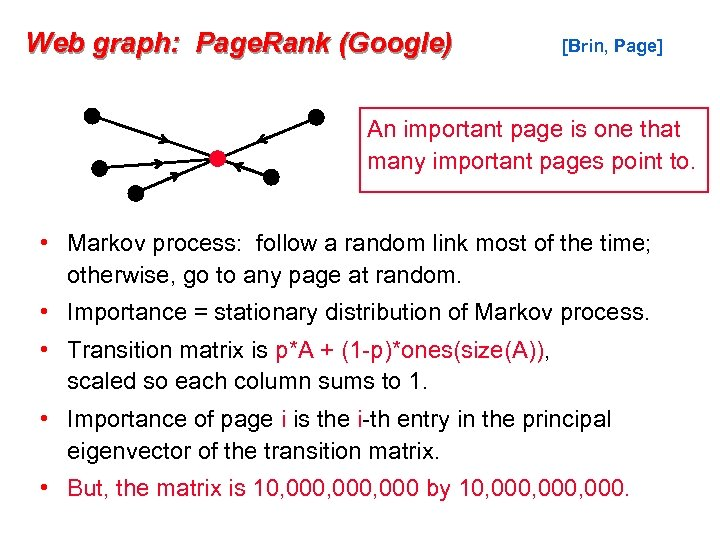Web graph: Page. Rank (Google) [Brin, Page] An important page is one that many important pages point to. • Markov process: follow a random link most of the time; otherwise, go to any page at random. • Importance = stationary distribution of Markov process. • Transition matrix is p*A + (1 -p)*ones(size(A)), scaled so each column sums to 1. • Importance of page i is the i-th entry in the principal eigenvector of the transition matrix. • But, the matrix is 10, 000, 000 by 10, 000, 000.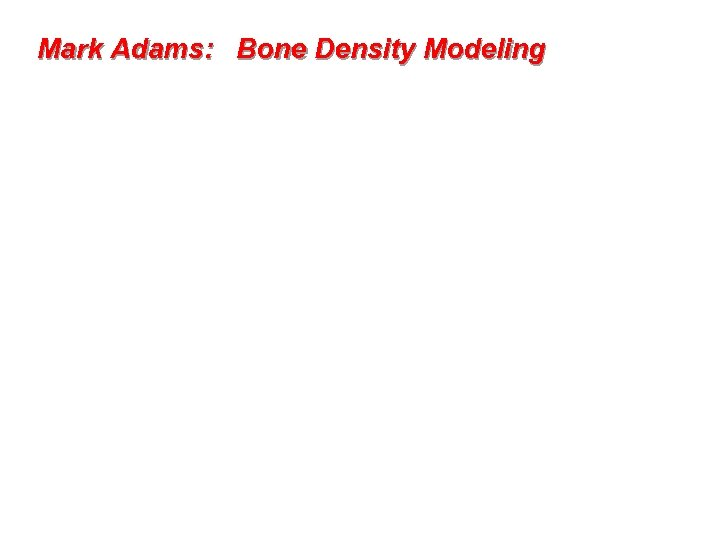Mark Adams: Bone Density Modeling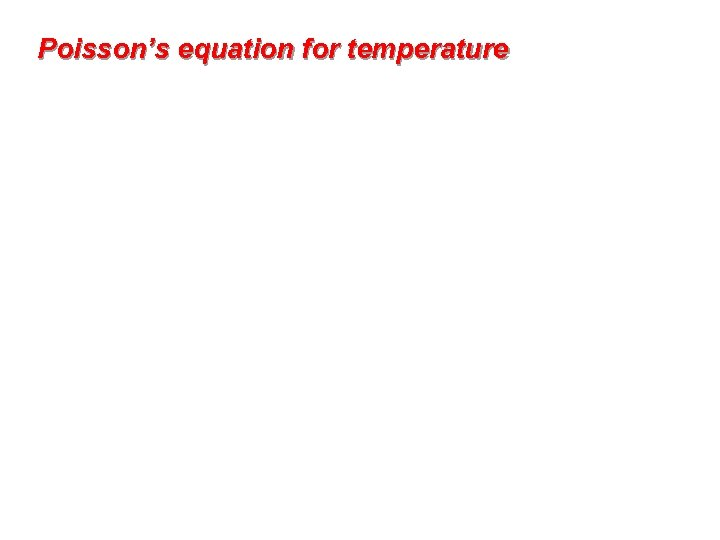Poisson’s equation for temperature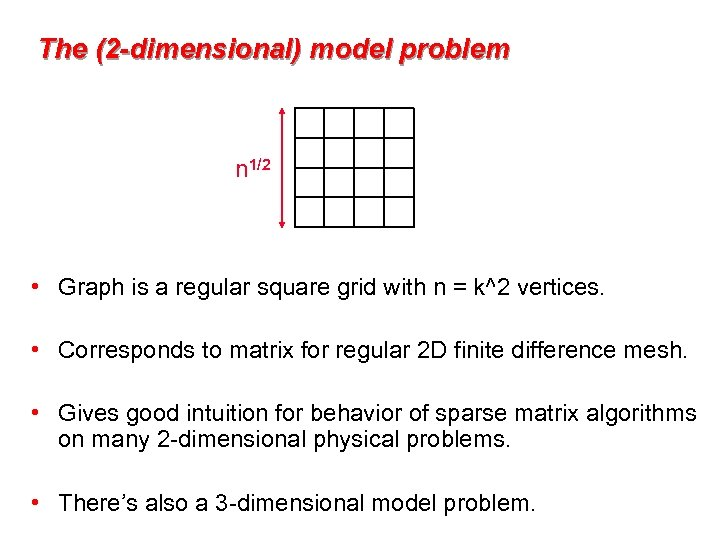The (2 -dimensional) model problem n 1/2 • Graph is a regular square grid with n = k^2 vertices. • Corresponds to matrix for regular 2 D finite difference mesh. • Gives good intuition for behavior of sparse matrix algorithms on many 2 -dimensional physical problems. • There’s also a 3 -dimensional model problem.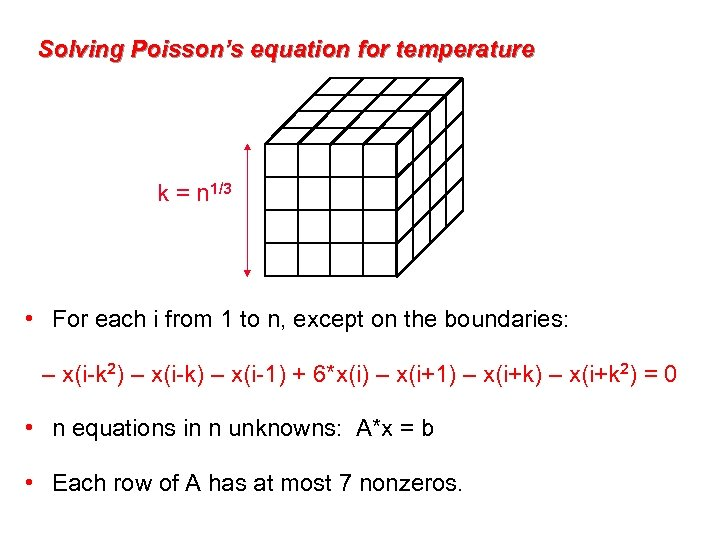Solving Poisson’s equation for temperature k = n 1/3 • For each i from 1 to n, except on the boundaries: – x(i-k 2) – x(i-k) – x(i-1) + 6*x(i) – x(i+1) – x(i+k 2) = 0 • n equations in n unknowns: A*x = b • Each row of A has at most 7 nonzeros.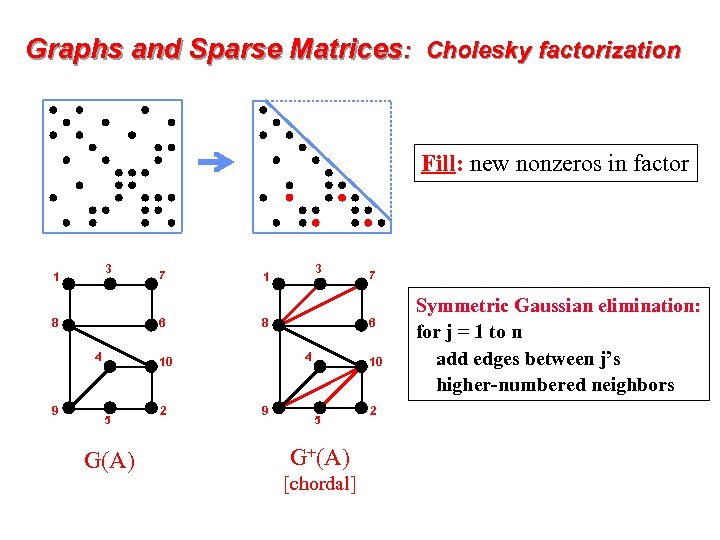Graphs and Sparse Matrices: Cholesky factorization Fill: new nonzeros in factor 3 1 6 8 4 9 7 G(A) 2 4 9 7 6 8 10 5 3 1 10 5 G+(A) [chordal] 2 Symmetric Gaussian elimination: for j = 1 to n add edges between j’s higher-numbered neighbors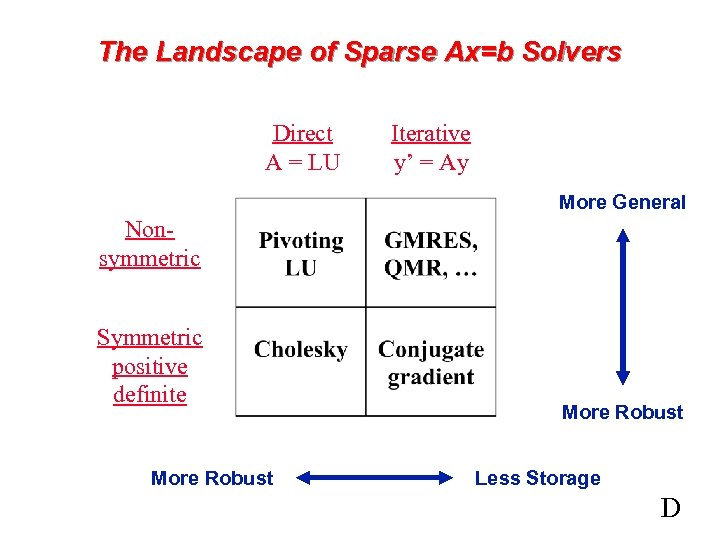The Landscape of Sparse Ax=b Solvers Direct A = LU Iterative y’ = Ay More General Nonsymmetric Symmetric positive definite More Robust Less Storage D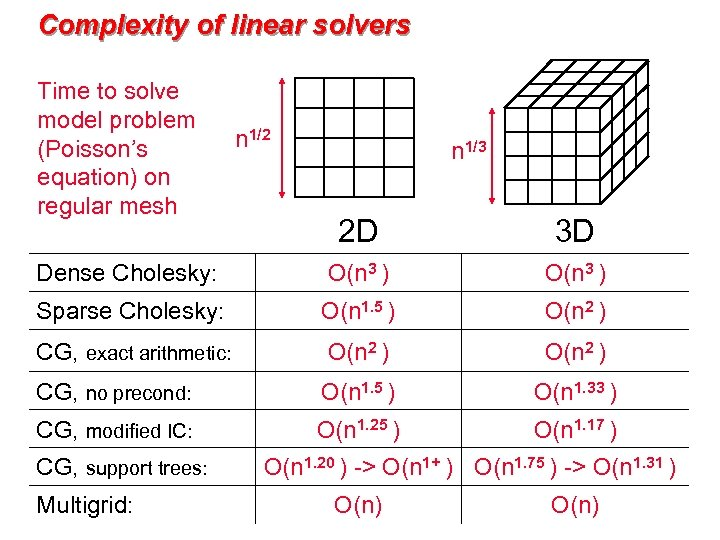Complexity of linear solvers Time to solve model problem (Poisson’s equation) on regular mesh n 1/2 n 1/3 2 D 3 D Dense Cholesky: O(n 3 ) Sparse Cholesky: O(n 1. 5 ) O(n 2 ) CG, exact arithmetic: O(n 2 ) CG, no precond: O(n 1. 5 ) O(n 1. 33 ) CG, modified IC: O(n 1. 25 ) O(n 1. 17 ) CG, support trees: Multigrid: O(n 1. 20 ) -> O(n 1+ ) O(n 1. 75 ) -> O(n 1. 31 ) O(n)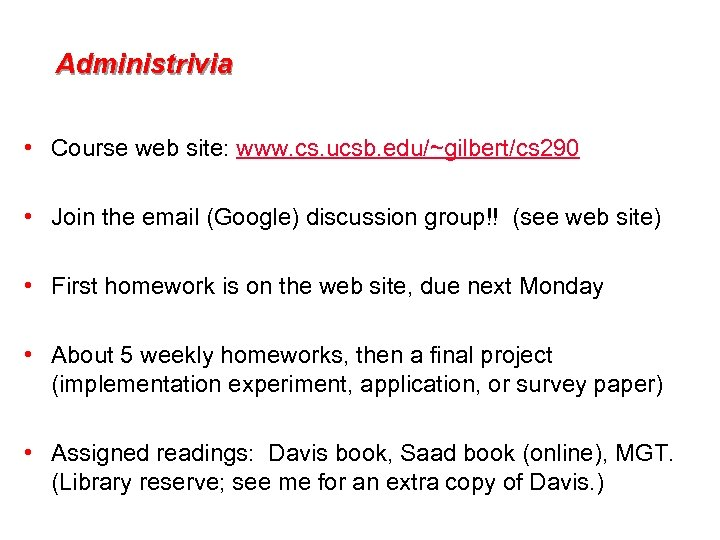Administrivia • Course web site: www. cs. ucsb. edu/~gilbert/cs 290 • Join the email (Google) discussion group!! (see web site) • First homework is on the web site, due next Monday • About 5 weekly homeworks, then a final project (implementation experiment, application, or survey paper) • Assigned readings: Davis book, Saad book (online), MGT. (Library reserve; see me for an extra copy of Davis. )